# Lecture 12: Coordinate Transformations, Recursion & Self-similarity II

## Annoucements

Assignment 06 Due Friday MONDAY!!!

• tester later this week

## Outline

1. Koch Curve
2. SVG Groups, Transformations, and Composition
3. Matrix Transformation Activity
4. Tree Example

## Motivation: Self-Similarity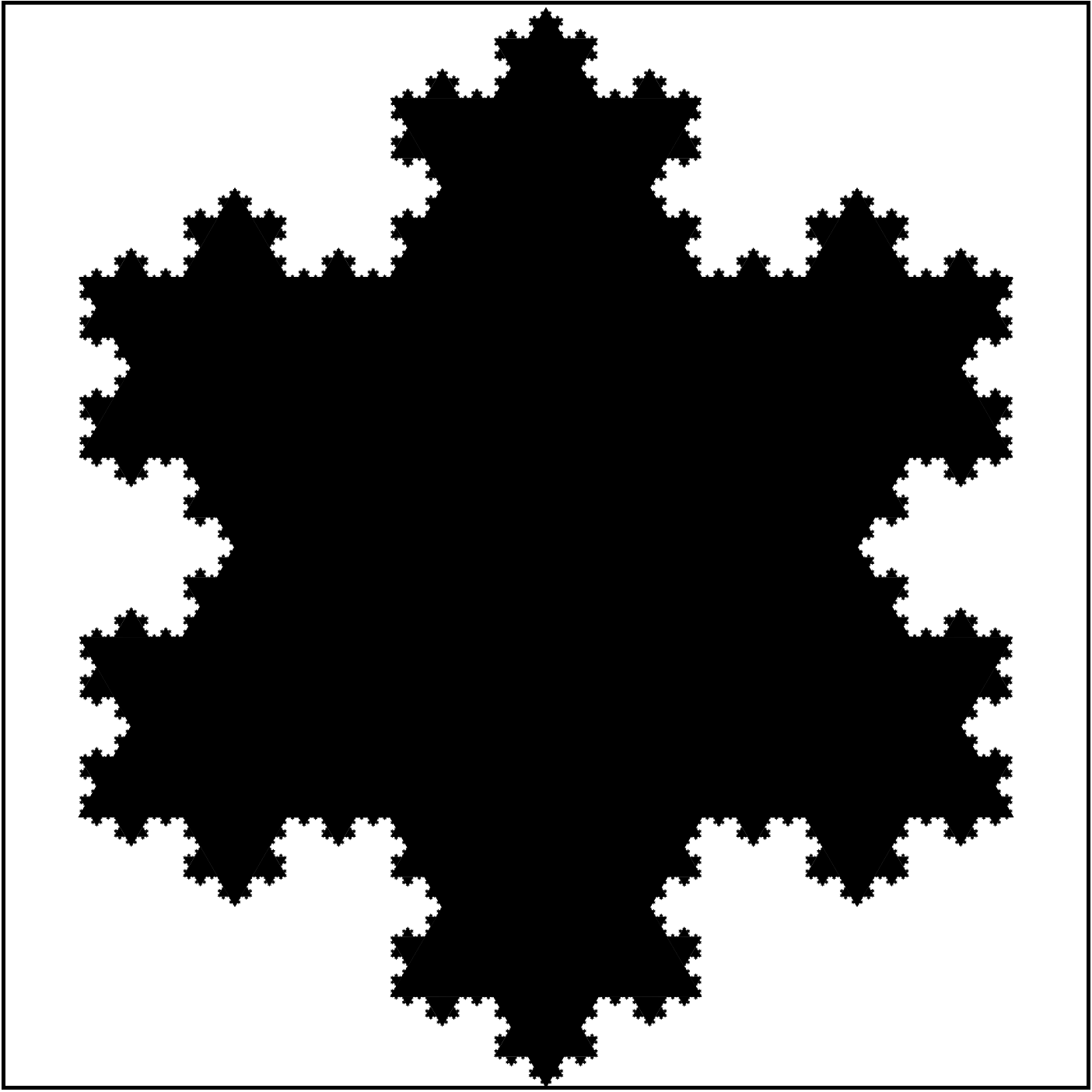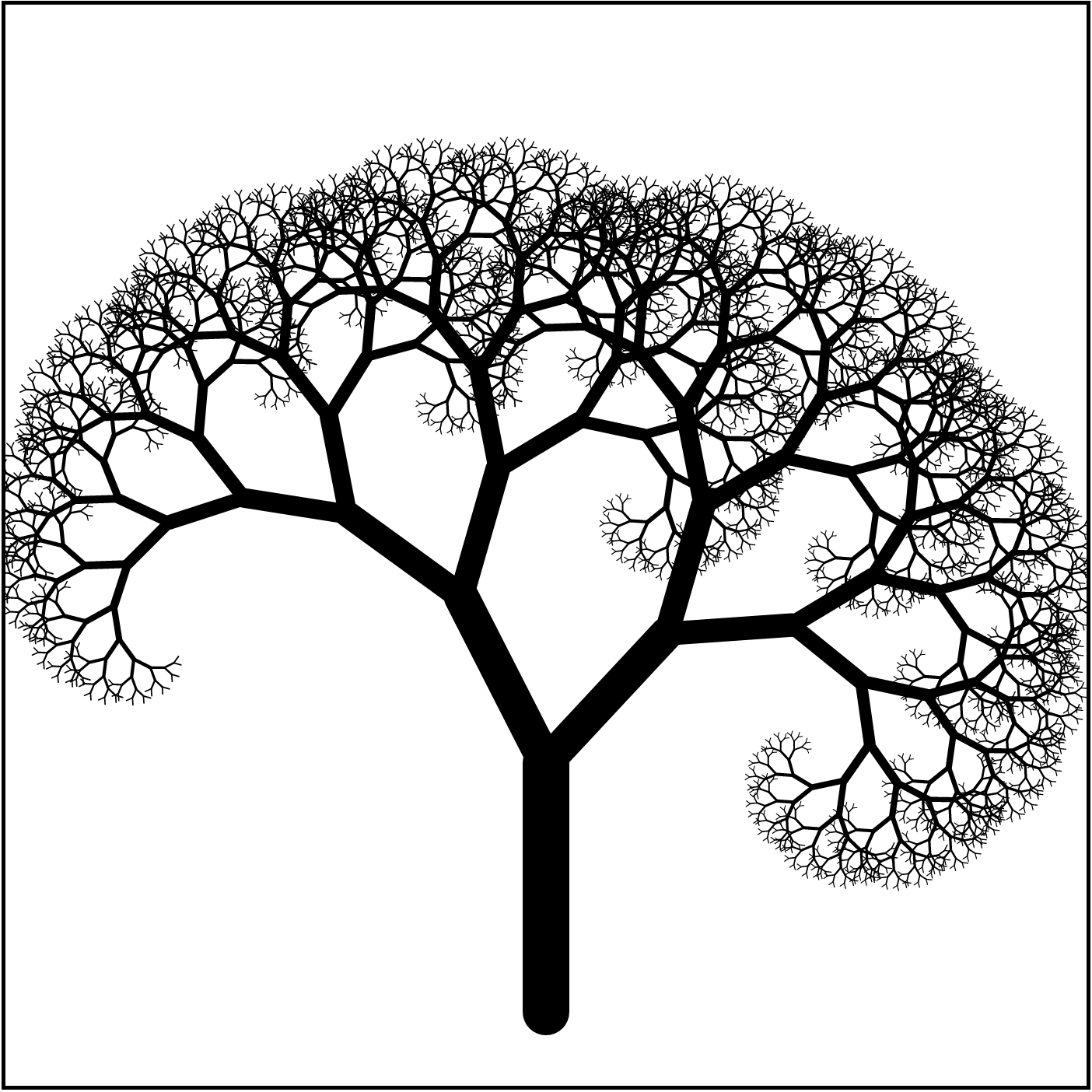## Example: Koch Curve I

How did we make the snowflake fractal?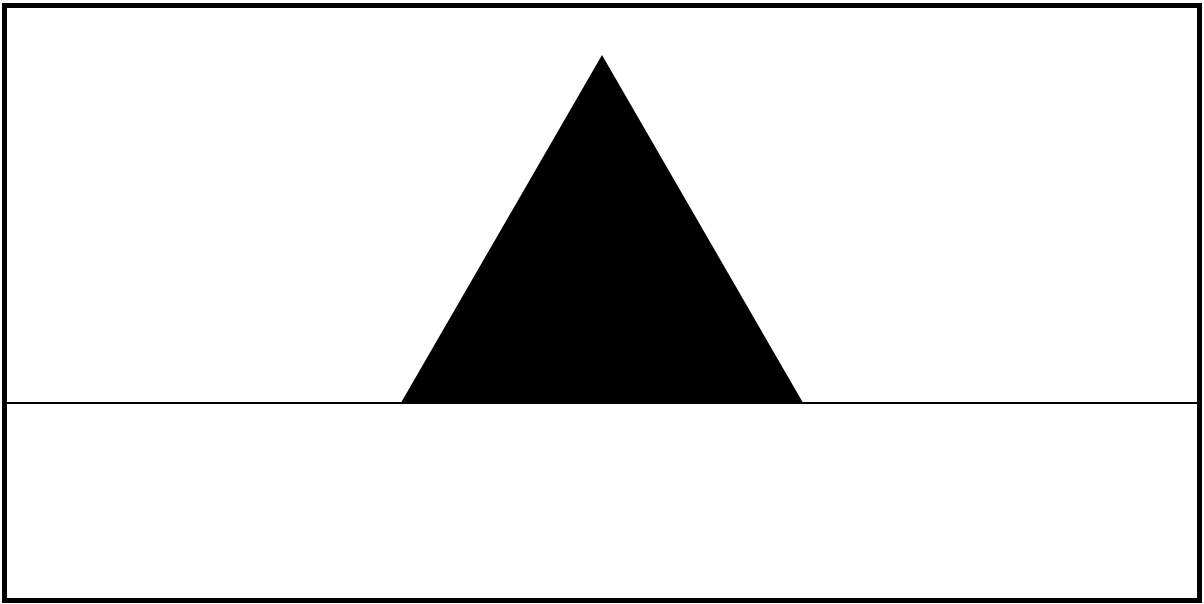Step 1: define a basic shape

## Example: Koch Curve II

How did we make the snowflake fractal?Step 2: define sub-shapes for basic shape

## Example: Koch Curve III

How did we make the snowflake fractal?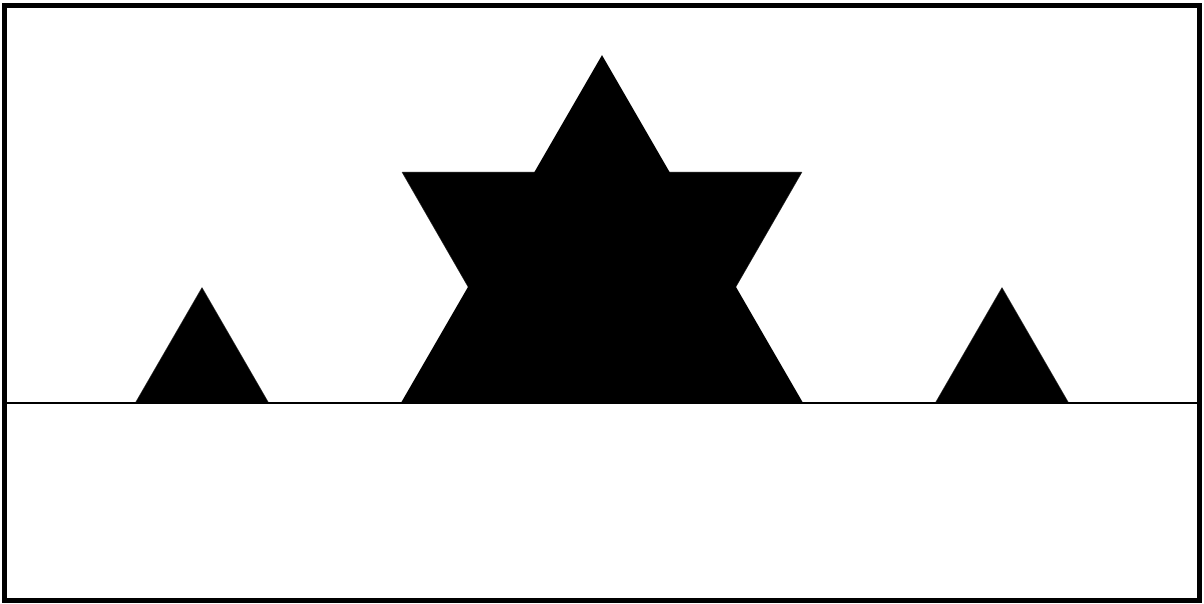Step 3: recurse

## Example: Koch Curve IV

How did we make the snowflake fractal?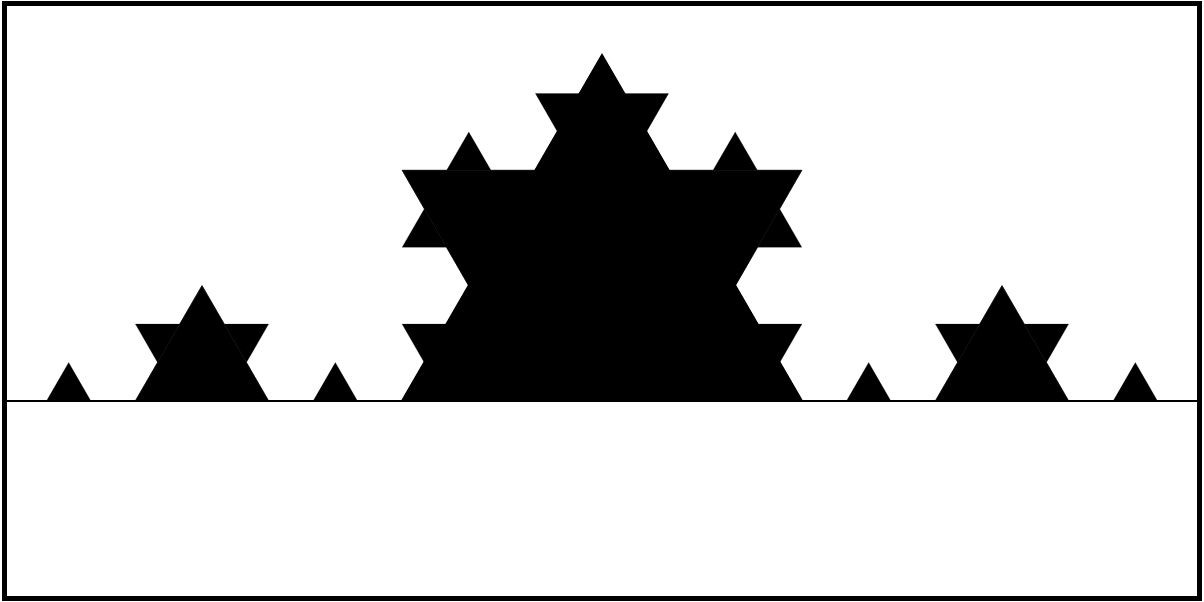Step 3: recurse

## ObservationEach iteration draws a bunch of transformed copies of the original shape

## Activity

Draw two iterations of the Koch curve!

• lec11-koch-step.zip

## The Basic ShapeIn koch.js:

• drawSegment(x1, y1, x2, y2) will draw the the basic shape transformed start at (x1, y1) and end at (x2, y2)
• in original, (x1, y1, x2, y2) = (0, 100, 600, 100),

## How to Add First Iteration?## The Second Iteration?## This Would Be Annoying!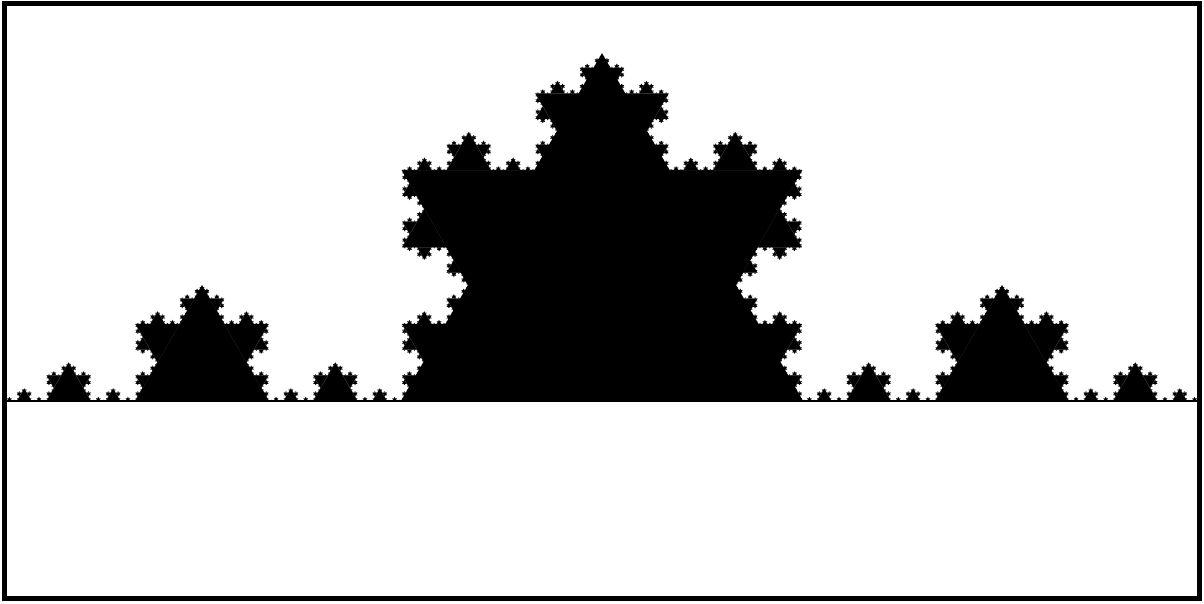## Composition

From last time: transformations compose

• perform transformation 1, then transformation 2
• transformation 2 is performed relative to transformation 1

## Transformed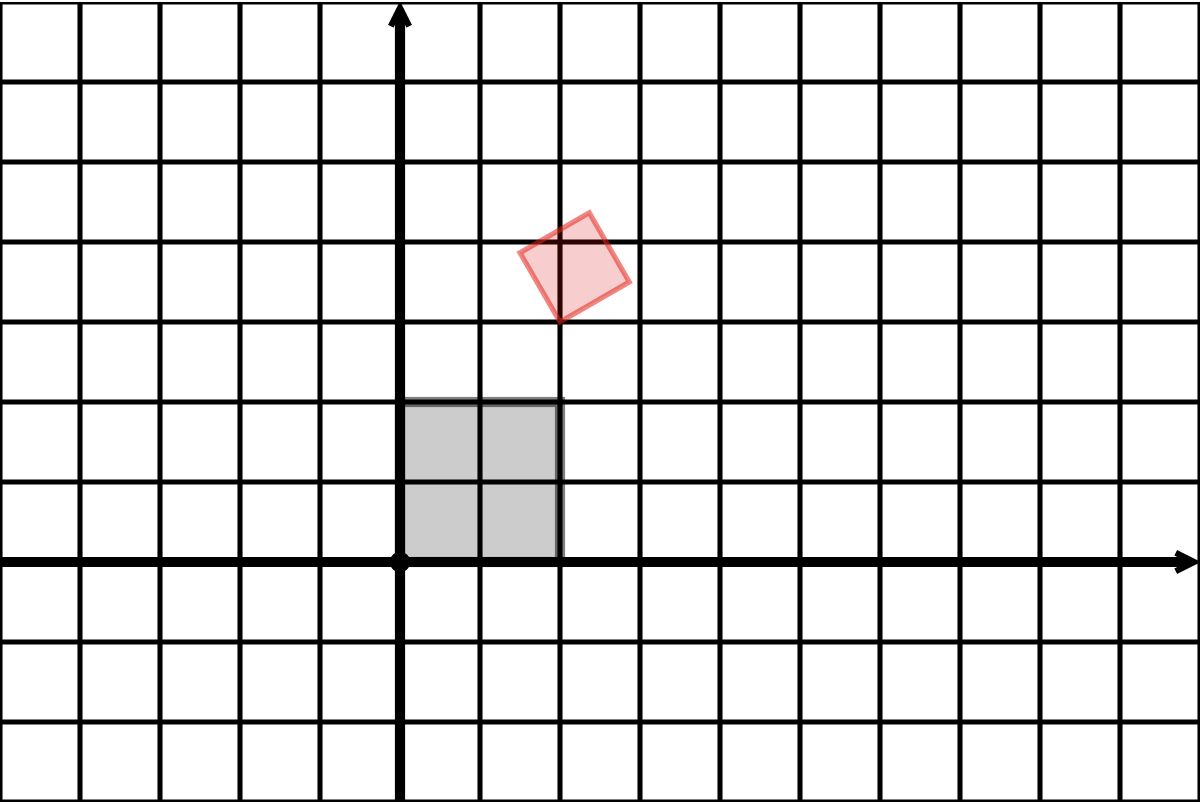## Transformed Coordinates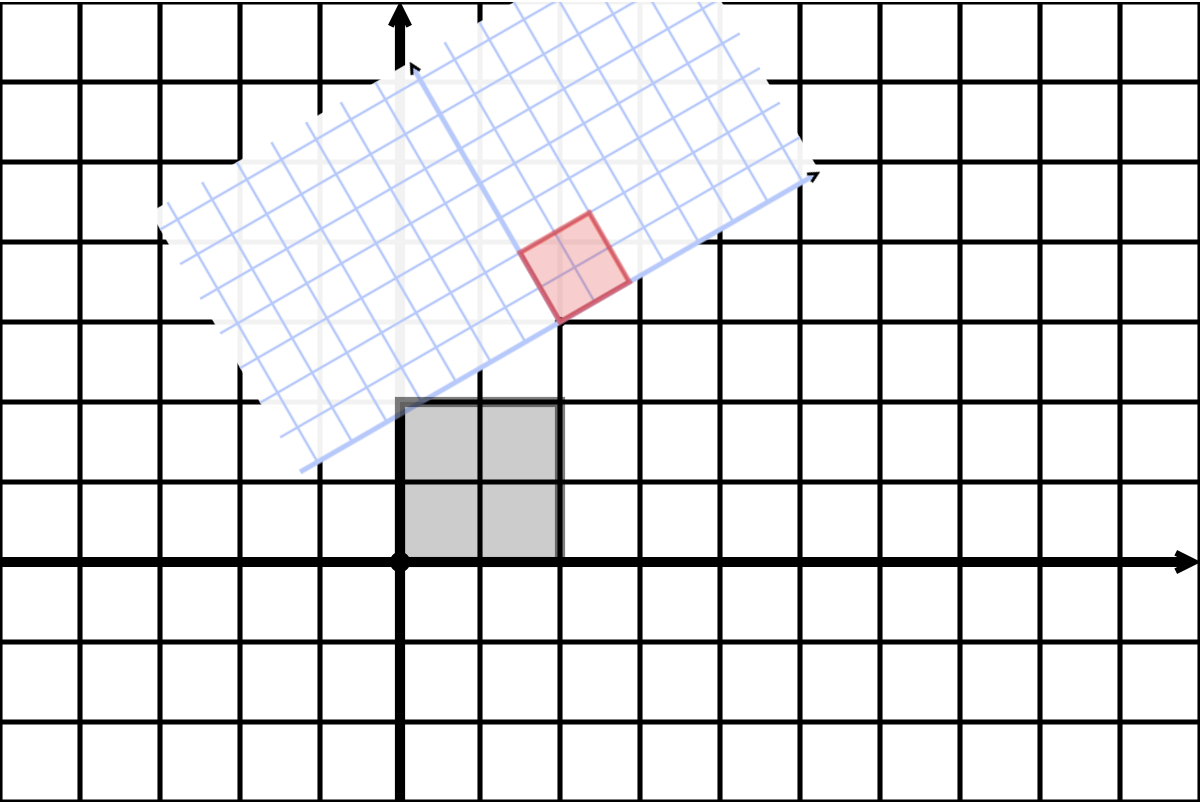## Element in New Coordinates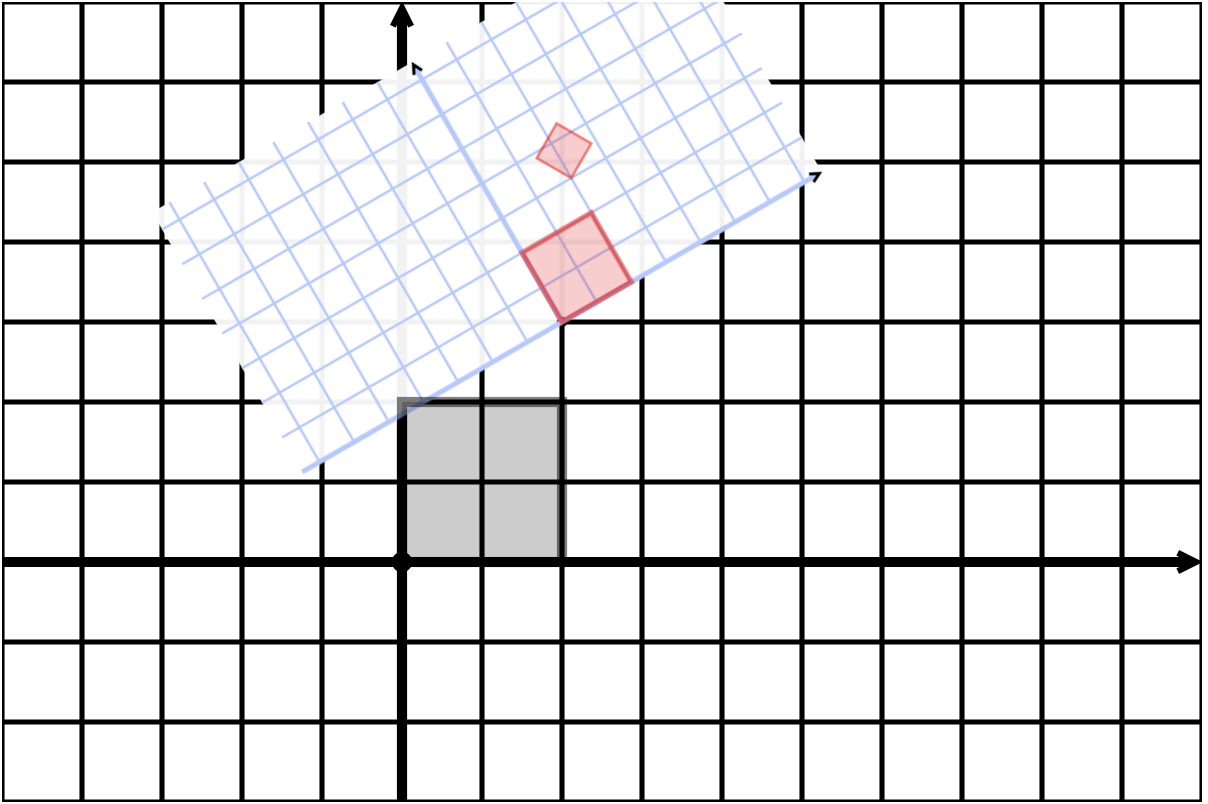## New New Coordinates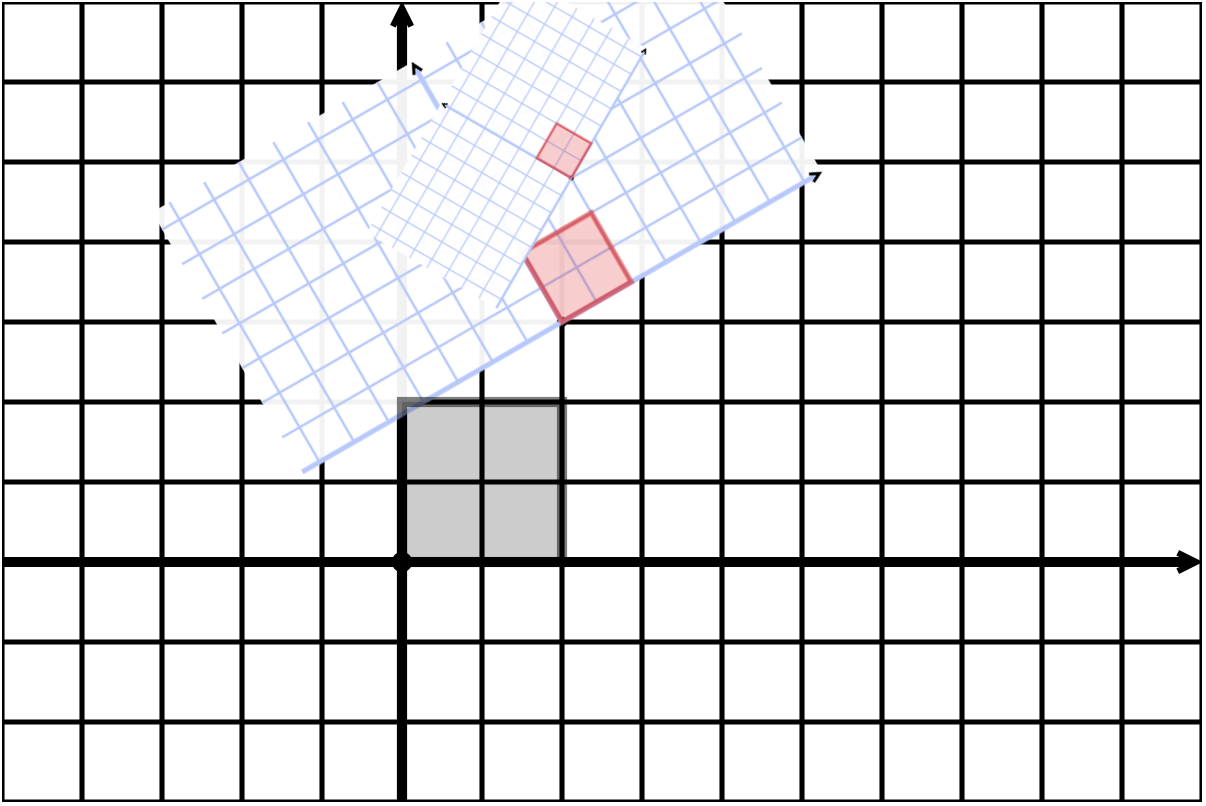## Koch Revisited

To draw a Koch segment:

1. Change to local coordinates for that segment
2. Draw a Koch segment
• relative to local coordinates instructions are the same as original segment
3. Recursively draw a Koch segment on each sub-segment

## Koch with Coordinates## The <g> Element

In SVG, <g> is a group element

• all elements in the same <g> are drawn together
• transformations of <g> are applied to all elements in the <g>
• <g> elements can be nested
• transformations of nested elements are composed

## Example

<rect width="20" height="20" fill="black">
<g transform="translate(30, 20) rotate(45)">
<rect width="20" height="20" fill="black">
<g transform="translate(30, 20)">
<rect width="20" height="20" fill="black">
</g>
</g>


## Drawing Koch Recursively

DrawKoch(parentGroup, transformation):
create and transform curGroup for this segment
draw this segment to curGroup
DrawKoch(curGroup, transformation for first sub-segment)
DrawKoch(curGroup, transformation for second sub-segment)
DrawKoch(curGroup, transformation for third sub-segment)
DrawKoch(curGroup, transformation for fourth sub-segment)


## Koch Demo

• lec12-koch-step.zip

## The matrix Reloaded

In SVG you can perform an affine transformation

• vector $(1, 0)$ to $(a, b)$
• vector $(0, 1)$ to $(c, d)$
• point $(0, 0)$ to $(e, f)$

with

transform=matrix(a, b, c, d, e, f)


Two squares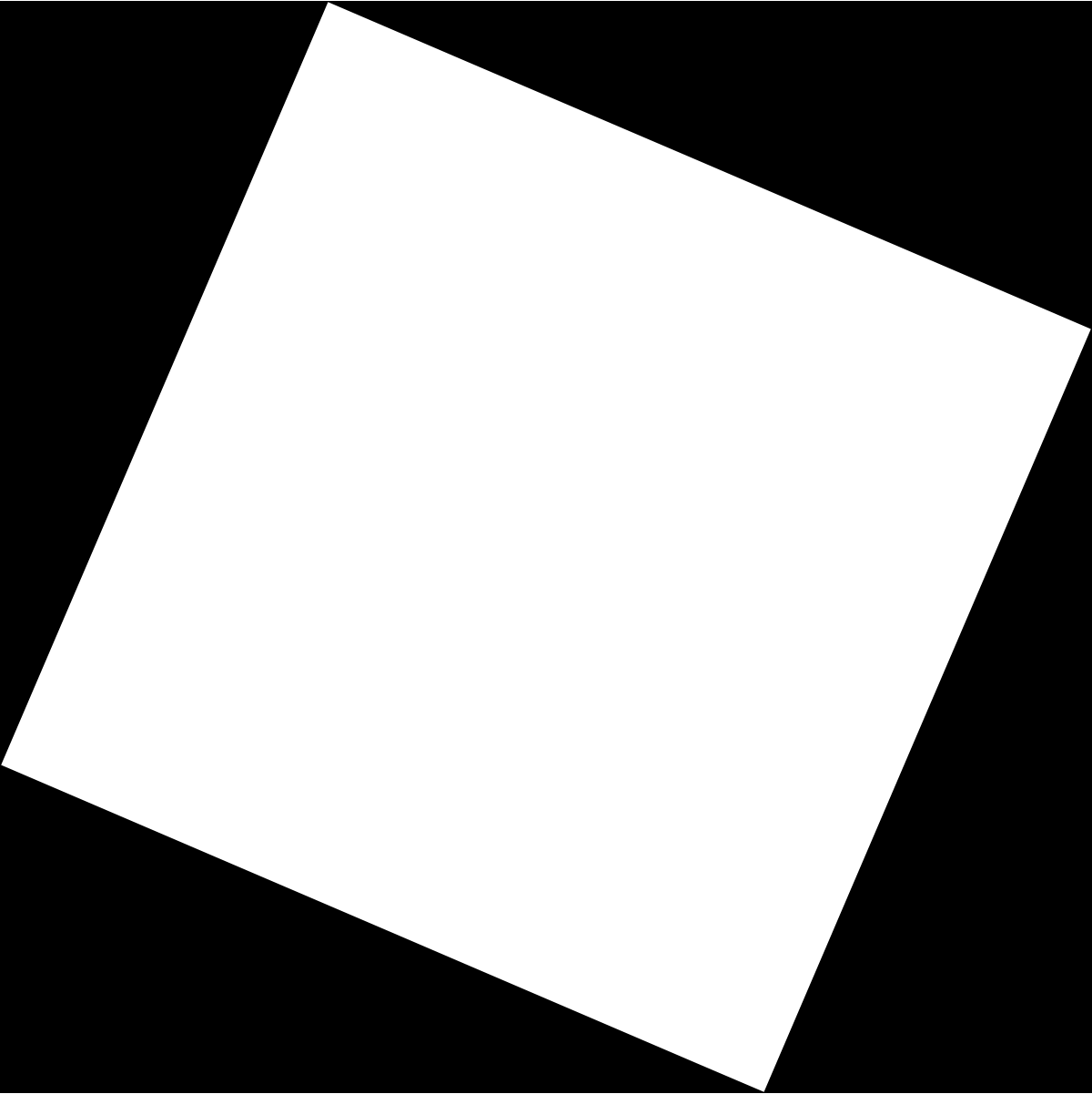## Activity: Find the matrixQuestion. What matrix transformation will transform the outer square to the inner square?

## How Did I Make This?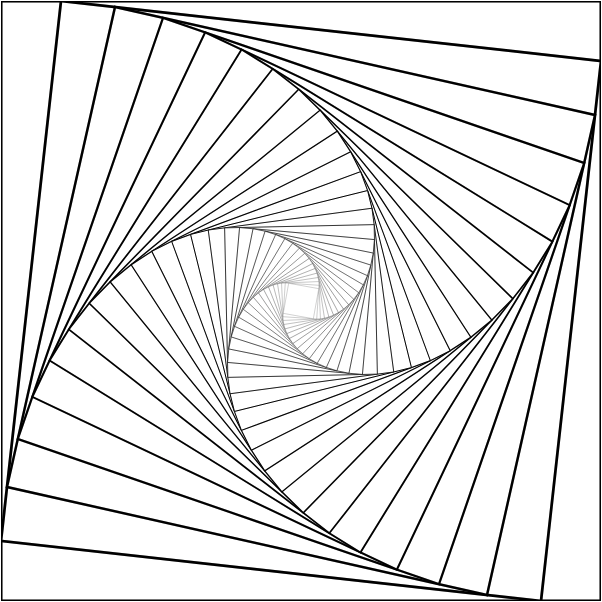## Recursive Squares Demo

• lec12-recusrive-squares.zip

## How Did I Make the Tree?## To Identify

1. Basic Shape

2. Recursive Step: transformation(s)?

## Recursive Tree Demo

• lec12-recursive-tree.zip

## Whoa, Dude

• lec12-animated-tree.zip

## Assignment 07

Make a website that incorporates (recursive) self-similar graphics

• must change some attribute by recursion depth

## Next Week

Automated Graph Drawing!

• Given (only) sets of vertices and edges of a graph, how can we draw it so that it looks nice?